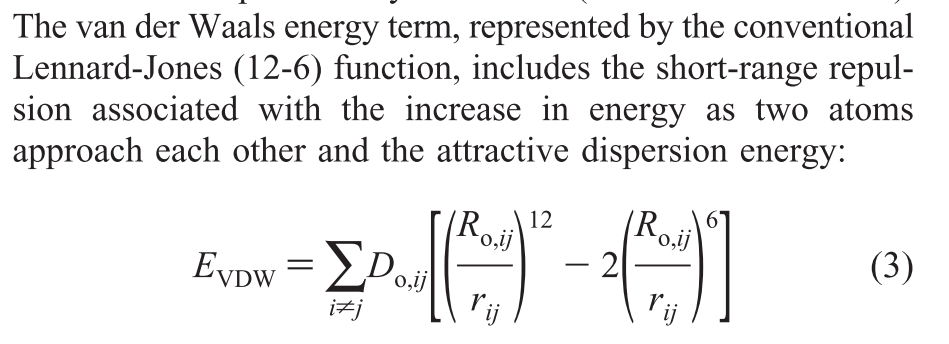# A question to modified lj potential

Dear all:
i want to simulate a Montmorillonite system and i find a paper that decipt a force field that exactly what i need.
but its pairwise potential confused me:i wonder which command or potential function i need to use to set this ?
THANK YOU!

Dear all:
i want to simulate a Montmorillonite system and i find a paper that decipt a force field that exactly what i need.
but its pairwise potential confused me:i wonder which command or potential function i need to use to set this ?

you can use the pair style lj/cut. the expression looks different due to the factor 2, but that is due to the different definition of the sigma parameter. there are two conventions for sigma: a) sigma is the radius where the LJ potential crosses 0, b) is the radius where the LJ potential is minimal. they differ by a factor of 2^(1/6) and thus you can easily convert the parameter.

axel.